# How To Model Volatility Smile In PythonIn this article, you will learn about the origin and implications of Volatility Smile. You will also see how to plot the volatility smile curve in Python. To start with, we will understand the concept of Volatility Smile by analyzing the assumption in Black Scholes Model (BSM), that the underlying’s daily returns are lognormally distributed.

### Black Scholes Model - Lognormal Assumption

BSM assumes the returns of the underlying to be lognormally distributed. Whereas in the real world it is observed that the distribution of returns is more complex than that. To understand this better, let us look at an example by analyzing the daily returns of the underlying as shown in the following table.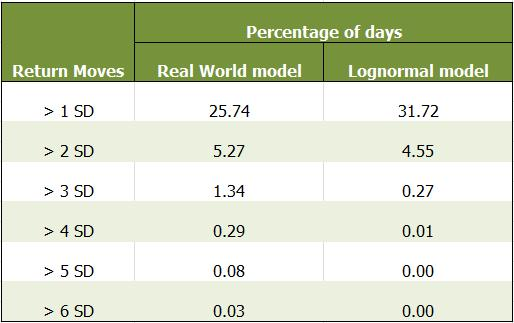The first column indicates the amount of deviation in daily returns on a given day, i.e. is the deviation in daily returns greater than one Standard Deviation, two standard deviations and so on till six standard deviations. The second and third columns store the percentage or probabilities of the daily returns to deviate more than one, two and so on till six standard deviations. The second column stores the values of a real asset, whereas the third column stores the values for an artificially created lognormal model.

Now, on comparing the values of percentages, it is observed that in the real world, there is a much higher percentage of the number of days when the returns values deviate more than 4, 5 and 6 standard deviations compared to the lognormal model. It can be seen that the percentage of values lying beyond 4 standard deviations is 29 times greater for real world assets than that for the lognormal model. Hence, we would like to do better than assume that the returns are lognormal.

### Volatility Smile

Deep out of the money options require higher deviations to end in the money. What this implies is that in the real world, the options that are deep out of the money are much more probable to end in the money when compared to an underlying that has a lognormal distribution. The BSM model calculates option premiums considering constant volatility and a lognormal distribution for the underlying. Hence the premiums computed using BSM will be cheaper as to what would be the fair value considering the underlying does not follow a lognormal distribution and does not have constant volatility. Therefore, when the values of call and put premiums are placed in the BSM formula to arrive at the value of implied volatility, it can be observed that the implied volatility is in the shape of a smile, with the minimum value for at the money options and volatilities rising as you move on either side as shown by the plot below.

### Plotting Volatility Smile in Python

Let us now understand how to plot the volatility smile in Python. Firstly, you need to see how the data is structured. Take a look at the dataframe below and observe the structure of the data, which has been slightly modified after downloading from NSE’s website for Nifty50 options.

#### 1. Importing Libraries

Now let us look at how to use this data for plotting the volatility smile. We will start by importing the relevant libraries.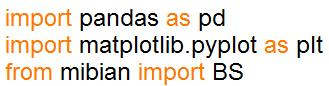We will import the pandas libraries to use the features of its powerful dataframe. Next, we will import the matplotlib.pyplot library for plotting the smile. Lastly, we will import the Mibian Library, which is used to compute the implied volatilities. We import the function BS from this library, which helps implement the BSM formula. To know more about this library and how it is used to carry out various computations, you may go through the latter half of the following blog post: Options Pricing Models – Black Scholes, Derman Kani & Heston Model

#### 2. Fetching Option Data

Next, we will fetch the option data that we have stored in a CSV file in the format shown above.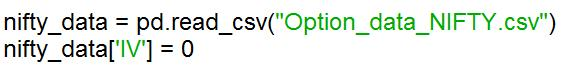We use the pandas library to convert the data in the csv file to a dataframe named nifty_data. We then create a new column for storing the implied volatilities under the column header ‘IV’ and initialize this column to store zeros.

#### 3. Calculating Implied Volatility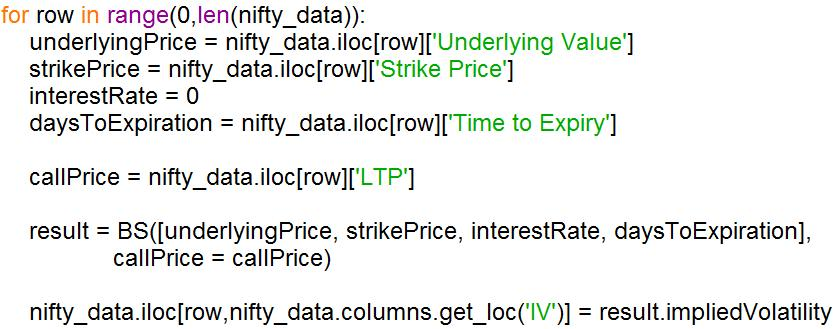We will now compute the implied volatilities on different dates for all the options in the dataframe nifty_data. We do so by running a for loop, iterating over all the rows of the dataframe nifty_data. We then define the different variables which will be used to call the BS function for computing the implied volatility. The interest rate is set to 0 because the value of the underlying in our dataframe is the value of the futures contract on Nifty50 and not the spot value of Nifty50. The values of implied volatility are calculated using the BS function as shown and stored in the dataframe nifty_data under the column header ‘IV’.

#### 4. Defining Plot_smile() function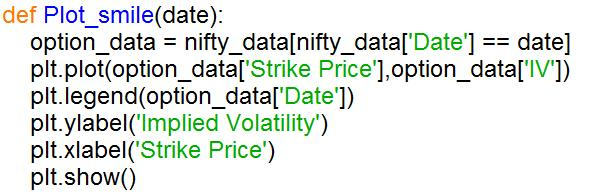Next, we define the Plot_smile() function which takes the date as input and plots the smile for that particular date. This is done by defining a new dataframe named option_data storing the rows of the dataframe nifty_data for that particular date. We then plot the smile by passing ‘Strike Price’ and ‘IV’ columns of option_data as x and y axis as arguments of the plot() function. We then define the legend and labels for the plot, finally showing the plot at the end of the function.

#### 5. Defining function to take input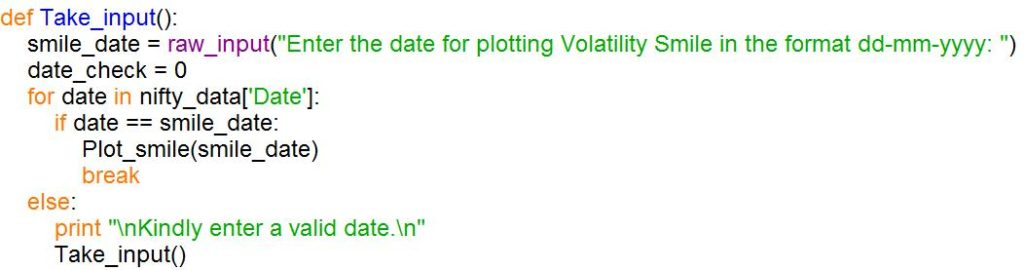#### 6. Calling the Take_input() function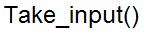Lastly, we call the Take_input() function for taking input from the user. The output plot from the code is shown below for 17th November, 2017.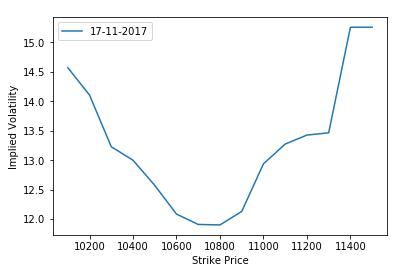### Conclusion

It is observed that the implied volatility curve is in the shape of a smile and is not flat as suggested by the assumption in BSM model. Therefore the underlying does not follow a lognormal distribution, but follows a modified random walk. The Derman Kani Model and Heston Models were developed to correct this false assumption in the BSM model.

If you are interested in learning to code trading strategies based on volatility, you should enrol for the following course on Quantra: